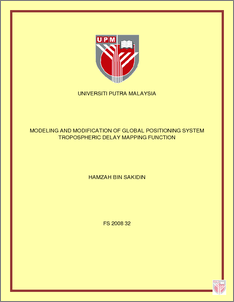Modeling and Modification of Global Positioning System Tropospheric Delay Mapping Function

Citation

Sakidin, Hamzah (2008) Modeling and Modification of Global Positioning System Tropospheric Delay Mapping Function. PhD thesis, Universiti Putra Malaysia.

Abstract

The accuracy of Global Positioning System (GPS) measurement is determined by the sum of several sources of error, such as orbit error, satellite clock error, multipath error, receiver noise error, selective availability, ephemeris error and also atmospheric error. The principal error source in the GPS technology is a delay experienced by the GPS signal in propagating through the electrically neutral atmosphere, usually referred to as a tropospheric delay. This delay is normally calculated in the zenith direction, and is referred to as a zenith tropospheric delay. The delay consists of a zenith hydrostatic delay, which can be modeled accurately using surface barometric measurements, and a zenith wet delay, which cannot be modeled from surface barometric measurements and depends on atmospheric water vapor. The mapping function is the coefficient for the zenith delay, either hydrostatic (dry) or non-hydrostatic (wet) delay that can be used to increase or reduce the tropospheric delay. In this research, 3 mapping function models which are known as, and Neill () are selected to be simplified, where as 2 mapping function models which are known as and Neill () are selected to be modified. For the simplification of the mapping function models, regression method has been used to find the suitable equation. The simplified mapping function models for, and Neill (), can reduce the computing time by reducing the percentage of number of operations between 71.4% to 92.3% for linear equations and 28.6% to 80.8% for quadratic equations. The calculations of the sum of errors show that the deviation of the simplified model from the original model is not significant. The simplification of the mapping function models can also create better understanding of the models by using hyperbolic, linear and also quadratic equations rather than continued fractions. Results indicate that the modification of the mapping function models can give smaller value especially for less than 5 degree elevation angles. As the coefficient of the zenith delay, it can improve the tropospheric delay directly. The improvement of the tropospheric delay for UNBabcand Neill mapping functions,can be obtained up to 19.1% and 17.8% respectively at 2 degree elevation angle.Preview
PDF
FS_2008_32.pdfView Item